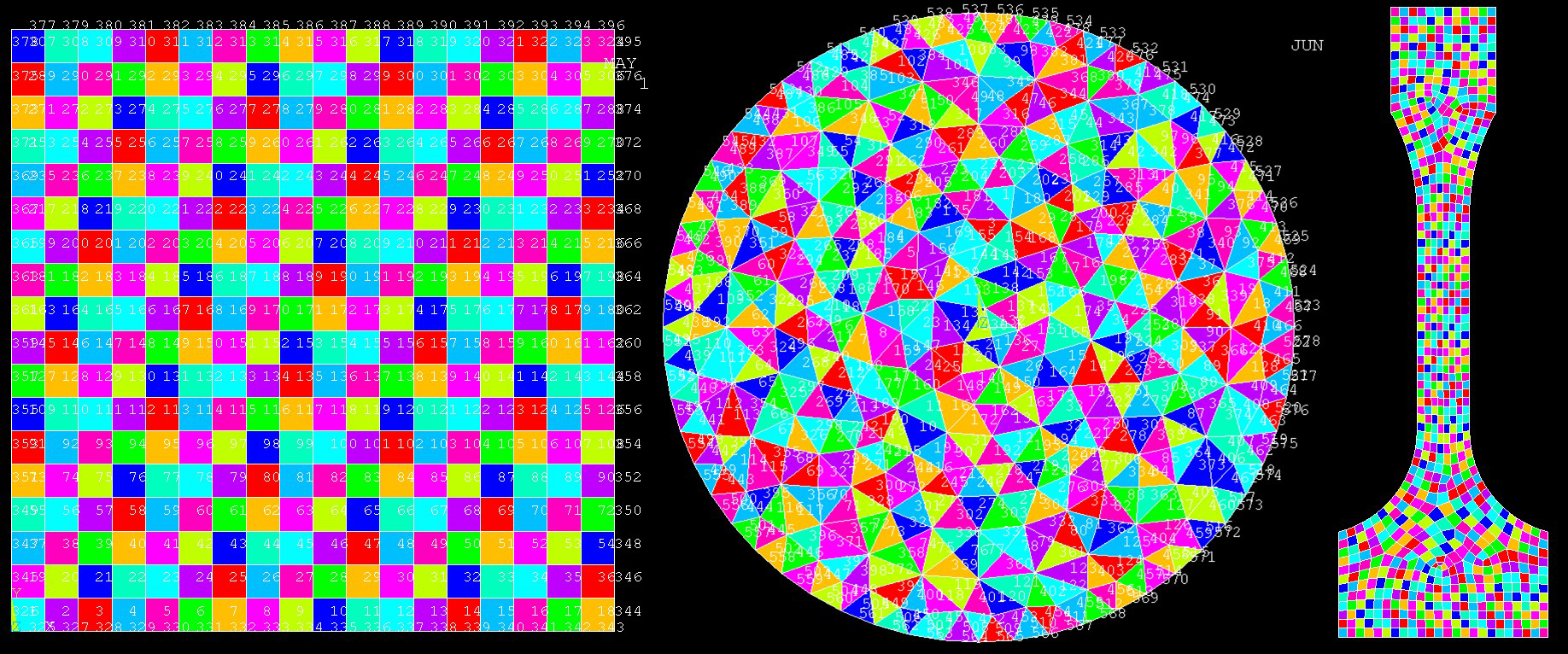# Surrogate Model Development for Turbocharger Life Prediction, 18-R8912

Principal Investigators
Grant Musgrove
Inclusive Dates
03/01/19 to 07/01/19

## Background

The current method to estimate turbocharger part life is to assume typical operating cycles of the turbocharger and predict the component stress and temperature values. These stress and temperature values are used with material fatigue data to estimate how many typical operating cycles the turbocharger can withstand. The problem with this generic approach is that most machinery does not always operate within the bounds of the typical cycle used to estimate part life. Therefore, schedule-based maintenance results in premature failure or premature repair. An unexpected failure or unneeded repair is a cost that turbomachinery operators would like to eliminate. The goal of this project was to increase the fidelity of the life prediction tool by developing the capability to identify turbocharger part life using actual engine operating data.

## Approach

A Finite Element Analysis (FEA) was used to predict the single-element temperatures of a simple 2D model. A thermal time constant was back-calculated at each time step based on the FEA results. This approach showed that the thermal time constant was not, in fact, constant, but actually increased with time. A relationship between the back-calculated thermal time constant and a physics-based thermal time constant was found, and a time-dependent thermal time constant was then calculated. This thermal time constant was used to predict element temperatures to various 2D model geometries using multiple heat transfer coefficient values. One predicted temperature was calculated for each time step over a period and then compared to the FEA results for each element that is at the surface of the model.Figure 1: Various shapes were created using FEA to predict surface element temperatures.

## Accomplishments

Temperature prediction results show a promising method to use for future work in component life predictions. The average percentage errors between the FEA results and the predicted results for the original geometry were all below the goal of 10%. Using the same logarithmic equations generated for the original geometry, thermal time constant values and predicted element temperatures were calculated for more complex geometry. As with the original geometry, the average percentage errors between the FEA results and the predicted results were all below the goal of 10%. This is an important result because it shows that the surface element temperatures on complex geometry can accurately be predicted using a thermal time constant calibrated to a simple 2D model.